Define PDE Boundary Condition VI

LabVIEW 2018 Help

Edition Date: March 2018
Part Number: 371361R-01
View Product InfoLabVIEW 2016 HelpLabVIEW 2017 HelpLabVIEW 2018 HelpLabVIEW 2019 HelpLabVIEW 2020 Help

Owning Palette: Partial Differential Equations VIs

Requires: Full Development System

Defines the boundary condition of partial differential equations. You must manually select the polymorphic instance to use.

Use the pull-down menu to select an instance of this VI.

 Select an instance 2D Helmholtz (Boundary Condition is Numeric)2D Helmholtz (Boundary Condition is VI)1D Heat (Boundary Condition is Numeric)1D Heat (Boundary Condition is VI)2D Heat (Boundary Condition is Numeric)2D Heat (Boundary Condition is VI)1D Wave (Boundary Condition is Numeric)1D Wave (Boundary Condition is VI)2D Wave (Boundary Condition is Numeric)2D Wave (Boundary Condition is VI)

2D Helmholtz (Boundary Condition is Numeric)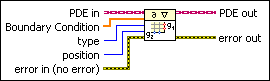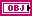PDE in is the class that stores the data of the equation.Boundary Condition specifies the value of the boundary condition.

If you define the equation on the rectangular domain, Boundary Condition stores the values of the unknown function evaluated at position. When position is Start X or End X, the length of Boundary Condition must be # of y-points from the Define PDE Domain VI. When position is Start Y or End Y, the length of Boundary Condition must be # of x-points from the Define PDE Domain VI. If the equation is defined on the polygonal domain, Boundary Condition stores the values of the unknown function evaluated at Boundary Points from the Define PDE Domain VI. The length of Boundary Condition must equal the number of Boundary Points. By default, LabVIEW assumes that the boundary condition values are zeros.type specifies the type of boundary condition. If you define the equation on the polygonal domain, type must be Dirichlet.

 0 Dirichlet (default)—The boundary of the domain evaluates the value of the unknown function to specify the boundary condition. 1 Neumann—The boundary of the domain evaluates the value of the normal derivative of the unknown function to specify the boundary condition.position specifies the position of the boundary condition. If you define the equation on the polygonal domain, position must be Start X.

 0 Start X (default)—LabVIEW evaluates the boundary condition with start x of the rectangular domain or Boundary Points of the polygonal domain from the Define PDE Domain VI. 1 End X—LabVIEW evaluates the boundary condition with end x from the Define PDE Domain VI. 2 Start Y—LabVIEW evaluates the boundary condition with start y from the Define PDE Domain VI. 3 End Y—LabVIEW evaluates the boundary condition with end y from the Define PDE Domain VI.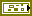error in describes error conditions that occur before this node runs. This input provides standard error in functionality.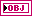PDE out returns PDE in with the boundary condition.error out contains error information. This output provides standard error out functionality.

2D Helmholtz (Boundary Condition is VI)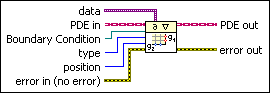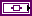data is a variant that passes arbitrary values to the VI.PDE in is the class that stores the data of the equation.Boundary Condition is a strictly typed reference to the VI that implements the boundary condition.

Create this VI by starting from the VI template located in labview\vi.lib\gmath\pde.llb\Common\2D Stationary PDE Func Template.vit.type specifies the type of boundary condition. If you define the equation on the polygonal domain, type must be Dirichlet.

 0 Dirichlet (default)—The boundary of the domain evaluates the value of the unknown function to specify the boundary condition. 1 Neumann—The boundary of the domain evaluates the value of the normal derivative of the unknown function to specify the boundary condition.position specifies the position of the boundary condition. If you define the equation on the polygonal domain, position must be Start X.

 0 Start X (default)—LabVIEW evaluates the boundary condition with start x of the rectangular domain or Boundary Points of the polygonal domain from the Define PDE Domain VI. 1 End X—LabVIEW evaluates the boundary condition with end x from the Define PDE Domain VI. 2 Start Y—LabVIEW evaluates the boundary condition with start y from the Define PDE Domain VI. 3 End Y—LabVIEW evaluates the boundary condition with end y from the Define PDE Domain VI.error in describes error conditions that occur before this node runs. This input provides standard error in functionality.PDE out returns PDE in with the boundary condition.error out contains error information. This output provides standard error out functionality.

1D Heat (Boundary Condition is Numeric)PDE in is the class that stores the data of the equation.Boundary Condition specifies the value of the boundary condition. Its length must be # of t-points from the Define PDE Domain VI. It stores the values of the unknown function evaluated at position over time. By default, LabVIEW assumes that the boundary condition values are zeros.type specifies the type of the boundary condition.

 0 Dirichlet (default)—The boundary of the domain evaluates the value of the unknown function to specify the boundary condition. 1 Neumann—The boundary of the domain evaluates the value of the normal derivative of the unknown function to specify the boundary condition.position specifies the position of the boundary condition.

 0 Start X (default)—LabVIEW evaluates the boundary condition with start x from the Define PDE Domain VI. 1 End X—LabVIEW evaluates the boundary condition with end x from the Define PDE Domain VI.error in describes error conditions that occur before this node runs. This input provides standard error in functionality.PDE out returns PDE in with the boundary condition.error out contains error information. This output provides standard error out functionality.

1D Heat (Boundary Condition is VI)data is a variant that passes arbitrary values to the VI.PDE in is the class that stores the data of the equation.Boundary Condition is a strictly typed reference to the VI that implements the boundary condition.

Create this VI by starting from the VI template located in labview\vi.lib\gmath\pde.llb\Common\1D Evolutionary PDE Func Template.vit.type specifies the type of the boundary condition.

 0 Dirichlet (default)—The boundary of the domain evaluates the value of the unknown function to specify the boundary condition. 1 Neumann—The boundary of the domain evaluates the value of the normal derivative of the unknown function to specify the boundary condition.position specifies the position of the boundary condition.

 0 Start X (default)—LabVIEW evaluates the boundary condition with start x from the Define PDE Domain VI. 1 End X—LabVIEW evaluates the boundary condition with end x from the Define PDE Domain VI.error in describes error conditions that occur before this node runs. This input provides standard error in functionality.PDE out returns PDE in with the boundary condition.error out contains error information. This output provides standard error out functionality.

2D Heat (Boundary Condition is Numeric)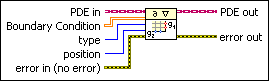PDE in is the class that stores the data of the equation.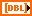Boundary Condition specifies the value of boundary condition.

When position is Start X or End X, the size of Boundary Condition must be # of t-points-by-# of y-points from the Define PDE Domain VI. Each row of Boundary Condition stores the values of the unknown function evaluated on points Y from the Define PDE Domain VI at a particular time step. Each column of Boundary Condition stores the values of the unknown function evaluated at a particular y-point over time. When position is Start Y or End Y, the size of Boundary Condition must be # of t-points-by-# of x-points from the Define PDE Domain VI. Each row of Boundary Condition stores the values of the unknown function evaluated on points X from the Define PDE Domain VI at a particular time step. Each column of Boundary Condition stores the values of the unknown function evaluated at particular x-points over time. By default, LabVIEW assumes that the boundary condition values are zeros.type specifies the type of the boundary condition.

 0 Dirichlet (default)—The boundary of the domain evaluates the value of the unknown function to specify the boundary condition. 1 Neumann—The boundary of the domain evaluates the value of the normal derivative of the unknown function to specify the boundary condition.position specifies the position of the boundary condition.

 0 Start X (default)—LabVIEW evaluates the boundary condition with start x from the Define PDE Domain VI. 1 End X—LabVIEW evaluates the boundary condition with end x from the Define PDE Domain VI. 2 Start Y—LabVIEW evaluates the boundary condition with start y from the Define PDE Domain VI. 3 End Y—LabVIEW evaluates the boundary condition with end y from the Define PDE Domain VI.error in describes error conditions that occur before this node runs. This input provides standard error in functionality.PDE out returns PDE in with the boundary condition.error out contains error information. This output provides standard error out functionality.

2D Heat (Boundary Condition is VI)data is a variant that passes arbitrary values to the VI.PDE in is the class that stores the data of the equation.Boundary Condition is a strictly typed reference to the VI that implements the boundary condition.

Create this VI by starting from the VI template located in labview\vi.lib\gmath\pde.llb\Common\2D Stationary PDE Func Template.vit.type specifies the type of the boundary condition.

 0 Dirichlet (default)—The boundary of the domain evaluates the value of the unknown function to specify the boundary condition. 1 Neumann—The boundary of the domain evaluates the value of the normal derivative of the unknown function to specify the boundary condition.position specifies the position of the boundary condition.

 0 Start X (default)—LabVIEW evaluates the boundary condition with start x from the Define PDE Domain VI. 1 End X—LabVIEW evaluates the boundary condition with end x from the Define PDE Domain VI. 2 Start Y—LabVIEW evaluates the boundary condition with start y from the Define PDE Domain VI. 3 End Y—LabVIEW evaluates the boundary condition with end y from the Define PDE Domain VI.error in describes error conditions that occur before this node runs. This input provides standard error in functionality.PDE out returns PDE in with the boundary condition.error out contains error information. This output provides standard error out functionality.

1D Wave (Boundary Condition is Numeric)PDE in is the class that stores the data of the equation.Boundary Condition specifies the value of the boundary condition. Its length must be # of t-points from the Define PDE Domain VI. It stores the values of the unknown function evaluated at position over time. By default, LabVIEW assumes that the boundary condition values are zeros.type specifies the type of the boundary condition.

 0 Dirichlet (default)—The boundary of the domain evaluates the value of the unknown function to specify the boundary condition. 1 Neumann—The boundary of the domain evaluates the value of the normal derivative of the unknown function to specify the boundary condition.position specifies the position of the boundary condition.

 0 Start X (default)—LabVIEW evaluates the boundary condition with start x from the Define PDE Domain VI. 1 End X—LabVIEW evaluates the boundary condition with end x from the Define PDE Domain VI.error in describes error conditions that occur before this node runs. This input provides standard error in functionality.PDE out returns PDE in with the boundary condition.error out contains error information. This output provides standard error out functionality.

1D Wave (Boundary Condition is VI)data is a variant that passes arbitrary values to the VI.PDE in is the class that stores the data of the equation.Boundary Condition is a strictly typed reference to the VI that implements the boundary condition.

Create this VI by starting from the VI template located in labview\vi.lib\gmath\pde.llb\Common\1D Evolutionary PDE Func Template.vit.type specifies the type of the boundary condition.

 0 Dirichlet (default)—The boundary of the domain evaluates the value of the unknown function to specify the boundary condition. 1 Neumann—The boundary of the domain evaluates the value of the normal derivative of the unknown function to specify the boundary condition.position specifies the position of the boundary condition.

 0 Start X (default)—LabVIEW evaluates the boundary condition with start x from the Define PDE Domain VI. 1 End X—LabVIEW evaluates the boundary condition with end x from the Define PDE Domain VI.error in describes error conditions that occur before this node runs. This input provides standard error in functionality.PDE out returns PDE in with the boundary condition.error out contains error information. This output provides standard error out functionality.

2D Wave (Boundary Condition is Numeric)PDE in is the class that stores the data of the equation.Boundary Condition specifies the value of boundary condition.

When position is Start X or End X, the size of Boundary Condition must be # of t-points-by-# of y-points from the Define PDE Domain VI. Each row of Boundary Condition stores the values of the unknown function evaluated on points Y from the Define PDE Domain VI at a particular time step. Each column of Boundary Condition stores the values of the unknown function evaluated at a particular y-point over time. When position is Start Y or End Y, the size of Boundary Condition must be # of t-points-by-# of x-points from the Define PDE Domain VI. Each row of Boundary Condition stores the values of the unknown function evaluated on points X from the Define PDE Domain VI at a particular time step. Each column of Boundary Condition stores the values of the unknown function evaluated at particular x-points over time. By default, LabVIEW assumes that the boundary condition values are zeros.type specifies the type of the boundary condition.

 0 Dirichlet (default)—The boundary of the domain evaluates the value of the unknown function to specify the boundary condition. 1 Neumann—The boundary of the domain evaluates the value of the normal derivative of the unknown function to specify the boundary condition.position specifies the position of the boundary condition.

 0 Start X (default)—LabVIEW evaluates the boundary condition with start x from the Define PDE Domain VI. 1 End X—LabVIEW evaluates the boundary condition with end x from the Define PDE Domain VI. 2 Start Y—LabVIEW evaluates the boundary condition with start y from the Define PDE Domain VI. 3 End Y—LabVIEW evaluates the boundary condition with end y from the Define PDE Domain VI.error in describes error conditions that occur before this node runs. This input provides standard error in functionality.PDE out returns PDE in with the boundary condition.error out contains error information. This output provides standard error out functionality.

2D Wave (Boundary Condition is VI)data is a variant that passes arbitrary values to the VI.PDE in is the class that stores the data of the equation.Boundary Condition is a strictly typed reference to the VI that implements the boundary condition.

Create this VI by starting from the VI template located in labview\vi.lib\gmath\pde.llb\Common\2D Stationary PDE Func Template.vit.type specifies the type of the boundary condition.

 0 Dirichlet (default)—The boundary of the domain evaluates the value of the unknown function to specify the boundary condition. 1 Neumann—The boundary of the domain evaluates the value of the normal derivative of the unknown function to specify the boundary condition.position specifies the position of the boundary condition.

 0 Start X (default)—LabVIEW evaluates the boundary condition with start x from the Define PDE Domain VI. 1 End X—LabVIEW evaluates the boundary condition with end x from the Define PDE Domain VI. 2 Start Y—LabVIEW evaluates the boundary condition with start y from the Define PDE Domain VI. 3 End Y—LabVIEW evaluates the boundary condition with end y from the Define PDE Domain VI.error in describes error conditions that occur before this node runs. This input provides standard error in functionality.PDE out returns PDE in with the boundary condition.error out contains error information. This output provides standard error out functionality.

Define PDE Boundary Condition Details

The following table gives the definitions of a normal derivative for one-dimensional equations and for two-dimensional equations defined on a rectangular domain.If the boundary type is Neumann, you must specify the value of the normal derivative of the unknown function and not the value of derivatives along the x- or y-axes. Moreover, you cannot specify the Neumann condition on a polygonal domain.
Position Normal Derivative (One-Dimension) Normal Derivative (Rectangular Domain)
Start X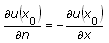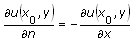End X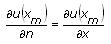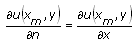Start Y N/A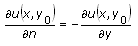End Y N/A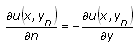The following block diagram is an example of defining the boundary condition for a one-dimensional wave equation. The boundary condition at Start X is Dirichlet, which the VI defines. The boundary condition at End X is Neumann, which the numeric array defines.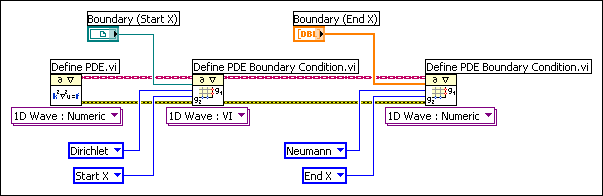Examples

Refer to the following VIs for examples of using the Define PDE Boundary Condition VI:

• PDE Flexible Element VI: labview\examples\Mathematics\Differential Equations - PDE
• PDE String Vibration VI: labview\examples\Mathematics\Differential Equations - PDE
• PDE Thermal Distribution VI: labview\examples\Mathematics\Differential Equations - PDE